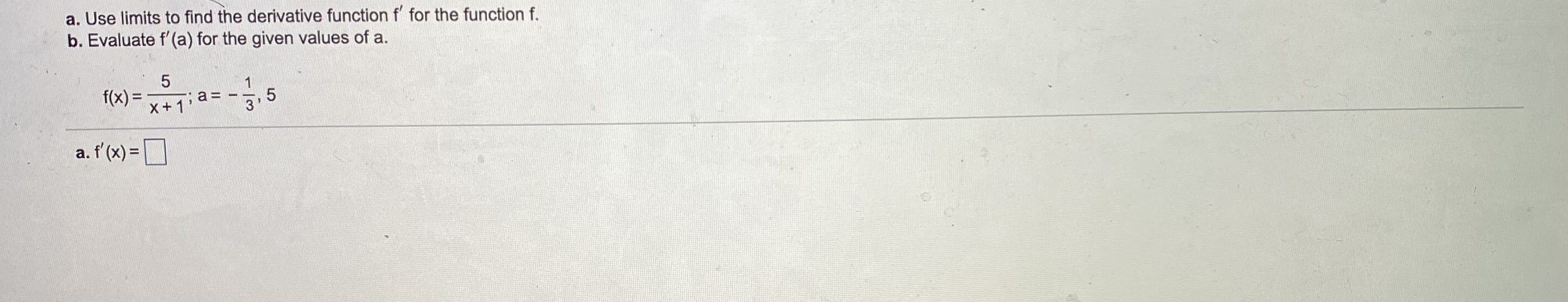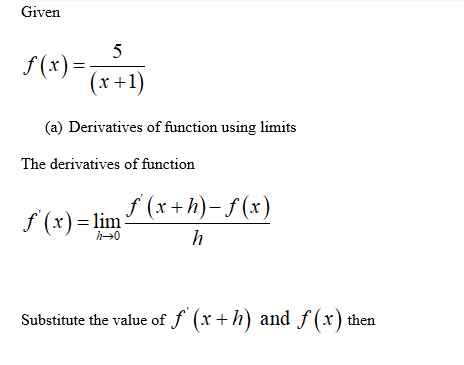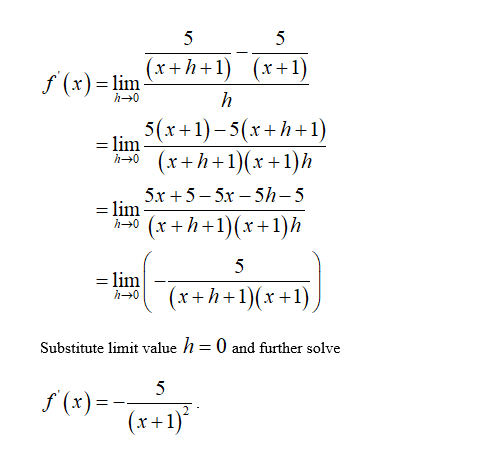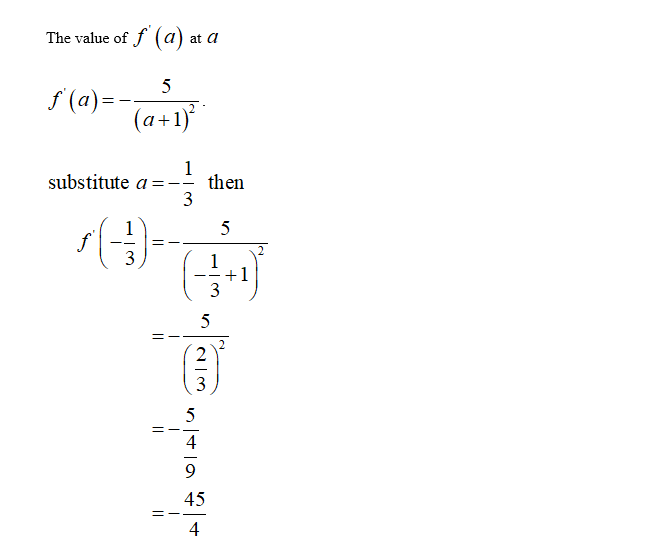# a. Use limits to find the derivative function f for the function f.b. Evaluate f'(a) for the given values of a.f(x) =,53X+1'a. f'(x) =D

Question
44 views

teacher covered this question in class, i was absent flu all weekhelp_outlineImage Transcriptionclosea. Use limits to find the derivative function f for the function f. b. Evaluate f'(a) for the given values of a. f(x) = ,5 3 X+1' a. f'(x) =D fullscreen
check_circle

Step 1Step 2Step 3...

### Want to see the full answer?

See Solution

#### Want to see this answer and more?

Solutions are written by subject experts who are available 24/7. Questions are typically answered within 1 hour.*

See Solution
*Response times may vary by subject and question.
Tagged in

### Calculus